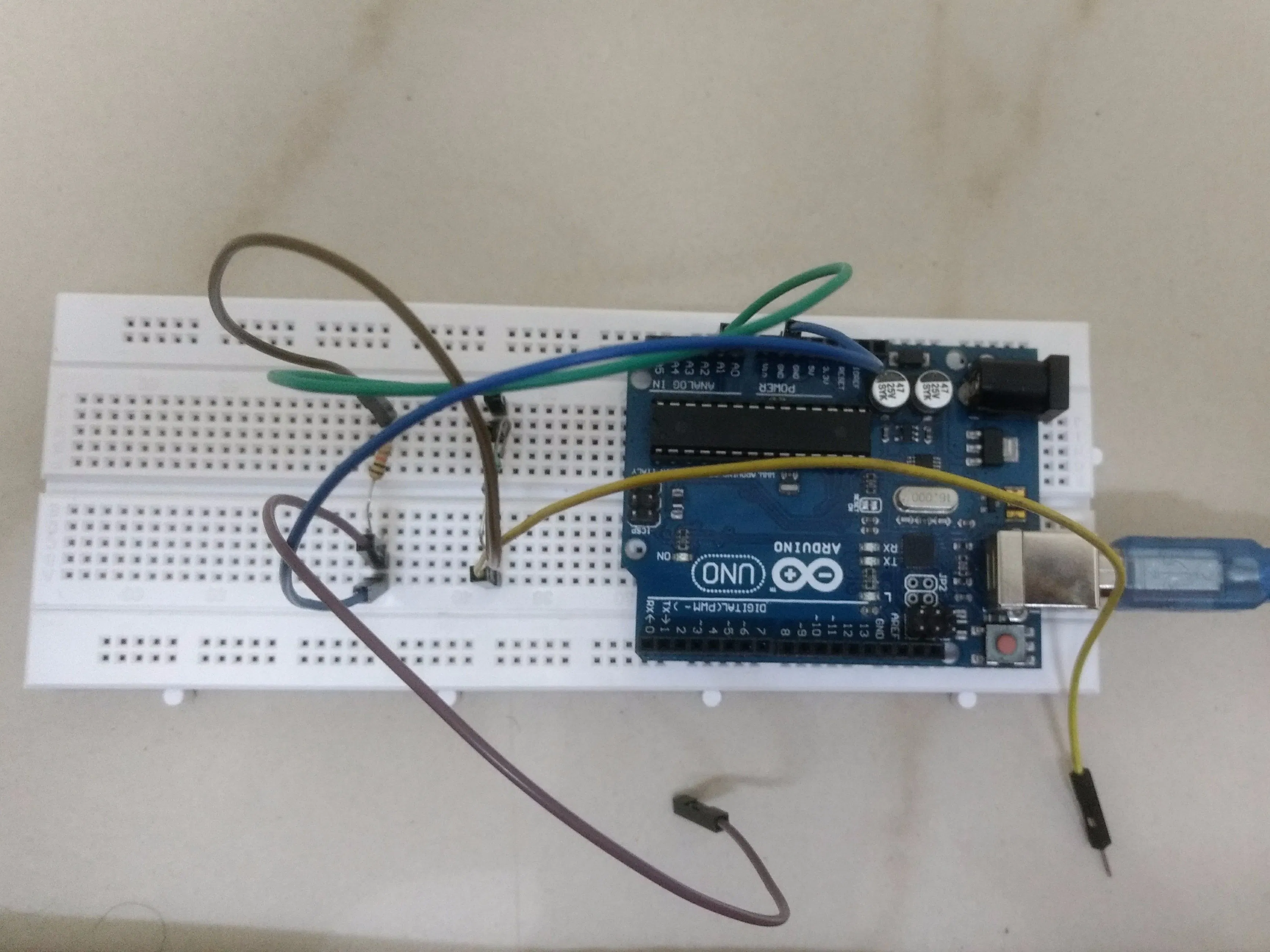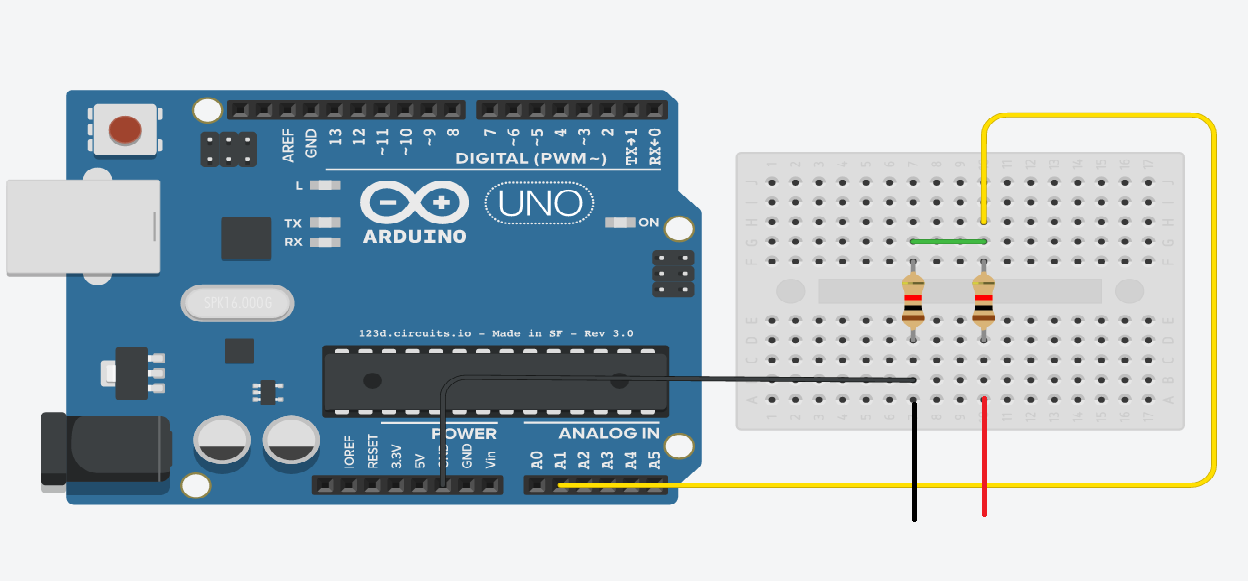Project showcase# Voltmeter Using Arduino © GPL3+

If you can't find a voltmeter at home or anywhere else, then this hack is for you!

• 70,668 views
• 92 respects

## Apps and online services

This project is about the simple hack of voltmeter by Arduino. The main reason behind making this is from my personal experience, when I was performing one experiment in the lab, I could not find a voltmeter so I made this hack to fulfill my requirement and also I think it will help others too.

Good project for beginners which uses basic concepts, yet a power full tool.

While running the code, you can find the output on the serial monitor.

Here, serial monitor will display the output i.e. Voltage.

We can also use different displays like LED Display for getting the output on it, instead of serial monitor. Finally, it depends on how you implement the project.

### Calculating Voltage

Formula for calculating voltage:

``````Vout = (Val * 5.0) / 1024.00;
``````

Here in these formula Val is the value that is read by Arduino as analog input, which is further multiplied by the voltage that is been supplied by Arduino and thus to get the Vout it is divided by the cycle of time that is covered after every bit to get the value.

``````Vin = Vout / (R2/R1+R2)
``````

By this formula we can find the Vin that will be around 0, which indirectly means we are creating ground.

NOTE: Here there is no restriction on using the specified amount of resistors, one can vary it according to the availability of the resistors.

If one has resistor of resistance say R ohm and other is R' ohm, then just one needs to change the const in the code.

Warning: Make sure that you don't mess with the anode and cathode part during testing, it may damage Arduino Board.

## Code

##### VoltmeterC/C++
Code for the project
```int analogInput = 1;
float Vout = 0.00;
float Vin = 0.00;
float R1 = 100000.00; // resistance of R1 (100K)
float R2 = 10000.00; // resistance of R2 (10K)
int val = 0;
void setup(){
pinMode(analogInput, INPUT); //assigning the input port
Serial.begin(9600); //BaudRate
}
void loop(){

Vout = (val * 5.00) / 1024.00; // formula for calculating voltage out i.e. V+, here 5.00
Vin = Vout / (R2/(R1+R2)); // formula for calculating voltage in i.e. GND
if (Vin<0.09)//condition
{
Vin=0.00;//statement to quash undesired reading !
}
Serial.print("\t Voltage of the given source = ");
Serial.print(Vin);
delay(1000); //for maintaining the speed of the output in serial moniter
}
```

## Schematics#### DIY Voltmeter with Arduino and a Nokia 5110 Display

Project tutorial by Nick Koumaris

• 17,132 views
• 23 respects

#### Arduino as a Voltmeter

Project tutorial by AJ-Explains-It-All

• 5,787 views
• 6 respects

#### Digital Voltmeter With Arduino Nano

Project showcase by KumarB

• 28,522 views
• 16 respects

#### Arduino Voltmeter (Standalone Application)

Project tutorial by abhinav

• 4,983 views
• 11 respects

#### Add WiFi to Arduino UNO

• 502,829 views